Over a million developers have joined DZone.{{announcement.body}}
{{announcement.title}}

DZone's Guide to

# Consensus Clustering Via Apache Spark

A look at Consensus Clustering to represent consensus across multiple runs of clustering algorithms and analyze the stability of the clusters with respect to sampling variability.

· Big Data Zone ·
Free Resource

Comment (0)

Save
{{ articles.views | formatCount}} Views

Cloudera Data Flow, the answer to all your real-time streaming data problems. Manage your data from edge to enterprise with a no-code approach to developing sophisticated streaming applications easily. Learn more today.

## Introduction

In this article, we will discuss a technique called Consensus Clustering to assess the stability of clusters generated by a clustering algorithm with respect to small perturbations in the data set. We will review a sample application built using the Apache Spark machine learning library to show how consensus clustering can be used with K-means, Bisecting K-means, and Gaussian Mixture, three distinct clustering algorithms.

Cluster analysis  in machine learning aims to partition data into separate, nonoverlapping sets based on a similarity measure between the data points. The data points in the same cluster must be as close (similar) to each other as possible and the data points in different clusters must be as distant (dissimilar) as possible. Cluster analysis has many applications in various scientific disciplines including biology, bioinformatics, medicine, business, computer science, and social sciences . Below are some examples.

• Clustering can be used for pixel classification of medical images in order to aid in medical diagnosis .

• Cancer patient data can be clustered into groups based on certain attributes, e.g. 'regional nodes positive' and 'stage group,' in order to analyze how life expectancy of patients varies depending on which cluster they belong to .

• Gene expressions of cancer cells can be profiled via clustering techniques in order to analyze cell structure and predict survival .

Many different techniques and algorithms are available for clustering analysis for which  and  provide excellent reviews.

### Problem Statement

Different clustering algorithms could create different clusters for the same data set. At different executions, even the same algorithm could produce different clusters, e.g. because of a random start. As an example, we downloaded a data set from the National Cancer Institute GDC Data Portal regarding Glioblastoma Multiforme (GBM) and Low-Grade Glioma (LGG) patients and partitioned them into three clusters using the K-means clustering algorithm.

The results are shown in Figures 1.a, 1.b for two different executions.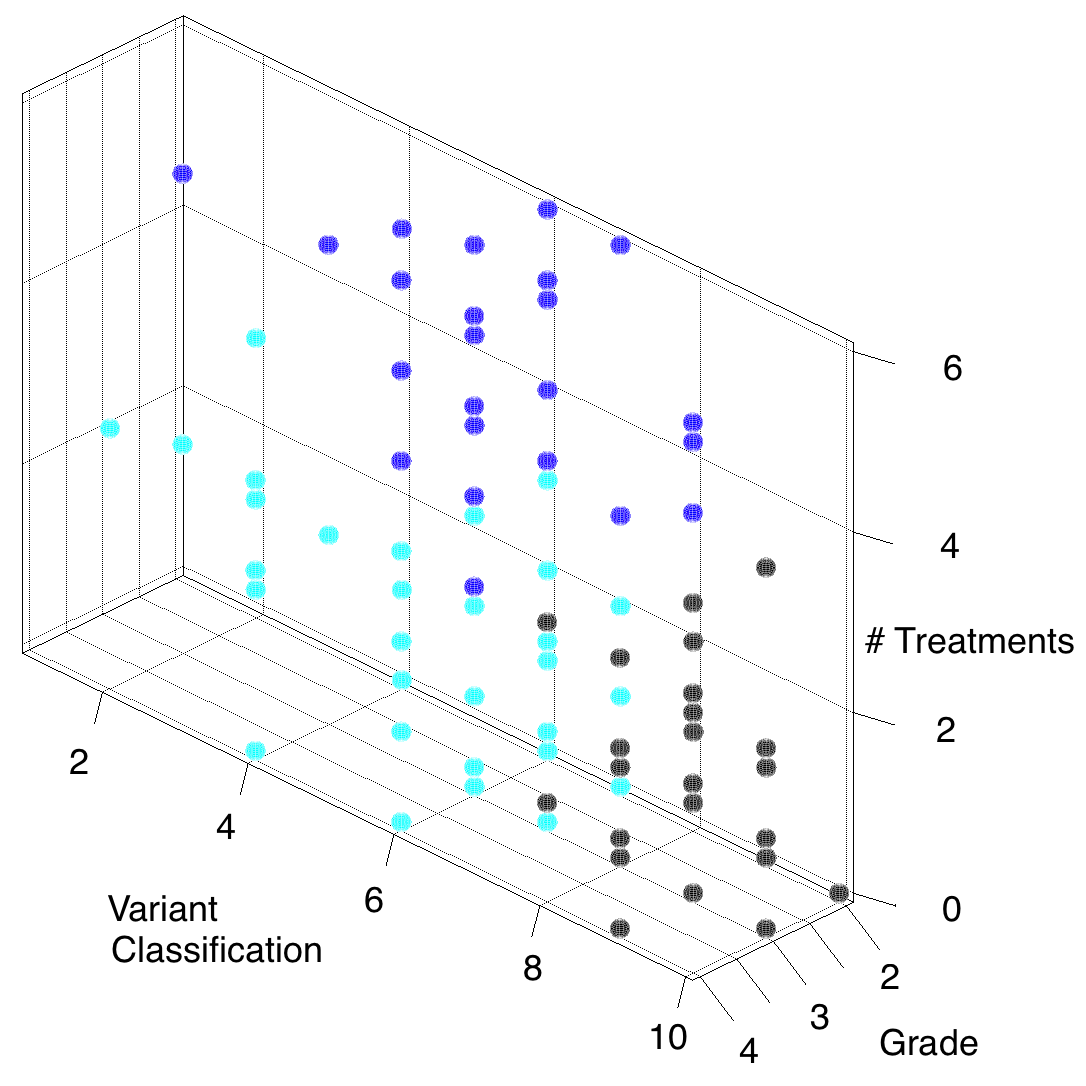Figure 1.a. A set of clusters obtained by K-means algorithm for GBM and LGG patients.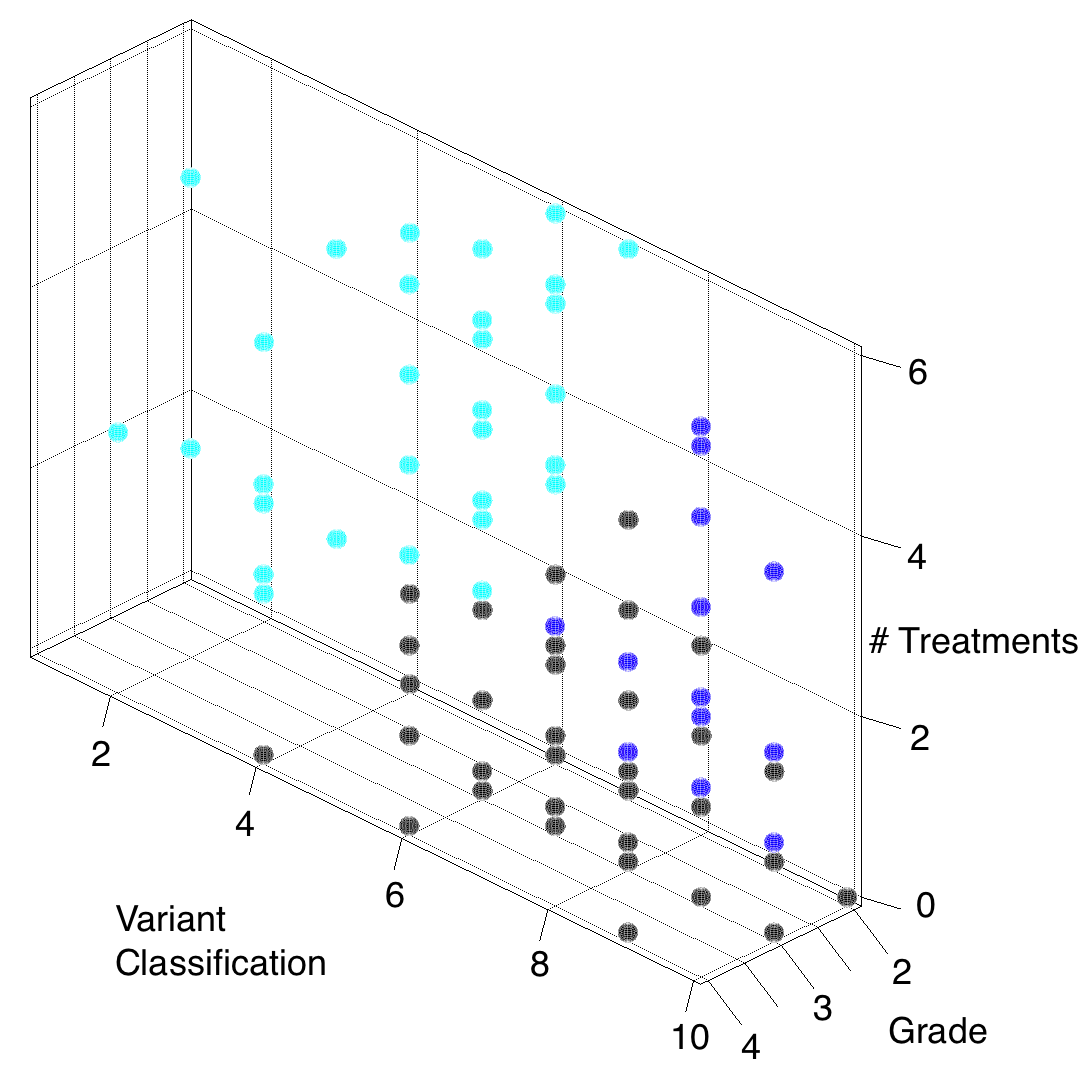Figure 1.b. Another set of clusters obtained by K-means algorithm for GBM and LGG patients.

In each diagram, there are three clusters depicted by black, dark blue, and light blue colors. The horizontal axes represent variant classification (a particular characteristic of the variant allele associated with the mutation of TP53 gene observed in the patient) and tumor grade. The vertical axis represents the number of chemotherapy treatments administered to the patient.

Notice that the cluster sets are not consistent. If an algorithm produces significantly different clusters for the same data set during different executions, can we trust that algorithm? How can we choose one algorithm over the other in order to feel most confident about the structure of clusters?

In this article, we will discuss an analytical technique called Consensus Clustering to represent consensus across multiple runs of a clustering algorithm and analyze the stability of discovered clusters with respect to sampling variability .

### Organization of Article

In the next section, we will discuss basics of consensus clustering. The following section is concerned with the code review of a sample application developed with the Apache Spark MLLib machine learning Library  to implement consensus clustering with three different clustering algorithms, K-means, Bisecting K-means, and Gaussian Mixture , . The last section gives conclusions.

## Consensus Clustering

The consensus clustering is based on the assumption that a reliable clustering algorithm should create similar clusters each time it is executed on a slightly different version of a data set. In other words, slight perturbations on a data set should not change the resulting clusters significantly.

In order to implement consensus clustering, the original data set is randomly subsampled m times to create m different data sets of slightly smaller size than the original one. Then the clustering algorithm is repeated m times (iterations), once for each subsampled data set.

Next, for each pair of data points in the original dataset, it is determined how many times the pair appears in the same cluster in a particular iteration of the clustering algorithm. The idea is that if the pair of data points are 'similar' they should ideally be placed in the same cluster in every iteration when the subsampled data set corresponding to the iteration contains both. Similarly, if the data points are 'dissimilar' they should be placed in distinct clusters in every iteration when the subsampled data set corresponding to the iteration contains both.

In order to quantify the consensus, a so-called 'consensus matrix' is constructed. Let the original data set consist of N data points. Then, the consensus matrix is a symmetric, N x N matrix where the element (i,j) is equal to the number of times data points ai and aj assigned into the same cluster divided by the number of times data points ai and aj were selected together. The diagonal elements of the consensus matrix are always 1 and any non-diagonal element is between 0 and 1, inclusive.

As a very simple example, consider a dataset consisting of 5 data points {a1, a2, a3, a4, a5} in 2-dimensional space.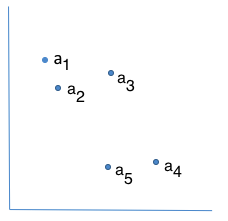Figure 2. An example of 5 data points in 2-dimensional space.

A consensus matrix for those data points could look like this:

 a1 a2 a3 a4 a5 a1 1 0.8 0.9 0.1 0 a2 0.8 1 0.7 0.05 0.01 a3 0.9 0.7 1 0.15 0.1 a4 0.1 0.05 0.15 1 0.9 a5 0 0.01 0.1 0.9 1

Consider the highlighted element (1,3) which corresponds to the pair of data points a1, a3. The value of that element implies that:

(# of times a1 and a3 are assigned to the same cluster) /
(# of times a1 and a3 selected together) = 0.9

Hence, 90% of the time when the points a1 and a3 were selected together in a data sample, the algorithm placed them in the same cluster. On the other hand, the consensus matrix implies that only 10% of the time the algorithm placed points a1 and a4 in the same cluster when they were selected together (element (1,4)).

A satisfactory consensus would imply that any non-diagonal element of the consensus matrix is either very close to 1, because the particular pair of data points are similar and therefore the algorithm assigned them to the same cluster in most iterations, or very close to 0, because the particular pair of data points are dissimilar and therefore the algorithm assigned them to distinct clusters in most iterations. On the other hand, entries that are away from both 0 and 1 imply poor consensus. For example, an entry with the value 0.5 implies that the algorithm has placed the corresponding pair of data points in the same cluster in 50% of the iterations and in separate clusters in the remaining 50% of the iterations.

The rows and columns of a consensus matrix could be permuted to place the closest data points as adjacent to each other as possible, producing a symmetric heat map, which then helps decide consensus via visual inspection . See  for a review of algorithms to produce a heat map from consensus matrix.

For visual inspection, an alternative approach to a heat map is to develop a histogram related to consensus matrix, called histogram of consensus indices . The horizontal axis of the histogram represents the ratio of how many times a pair of two distinct data points appeared in the same cluster to how many times that pair was in the same subsample across all iterations. The vertical axis of the histogram shows how many times the particular ratio is attained. In the ideal case the histogram will trend towards only two bins, near 1 and 0, where the bin near 1 represents the pair of distinct data points that were in the same cluster and the bin near 0 represents the pair of distinct data points that were in separate clusters most of the time across all iterations.

For each of the K-means, Bisecting K-means, and Gaussian Mixture algorithms, we applied a consensus clustering technique to our data set to create three clusters with m=20 iterations. In each iteration, the random subsample consisted of ~90% of the original dataset. The histograms for each algorithm are shown below (the source code of the application that generated those diagrams will be reviewed in the following section).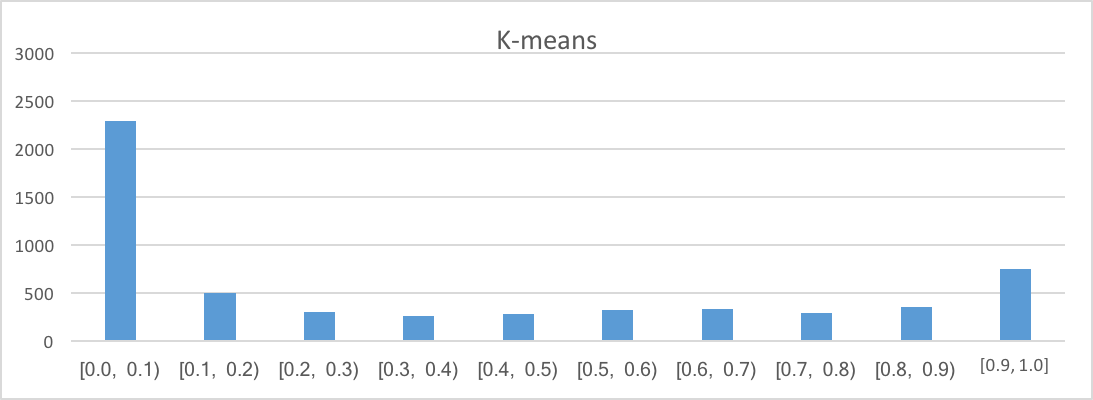Figure 3. Histogram for K-means algorithm.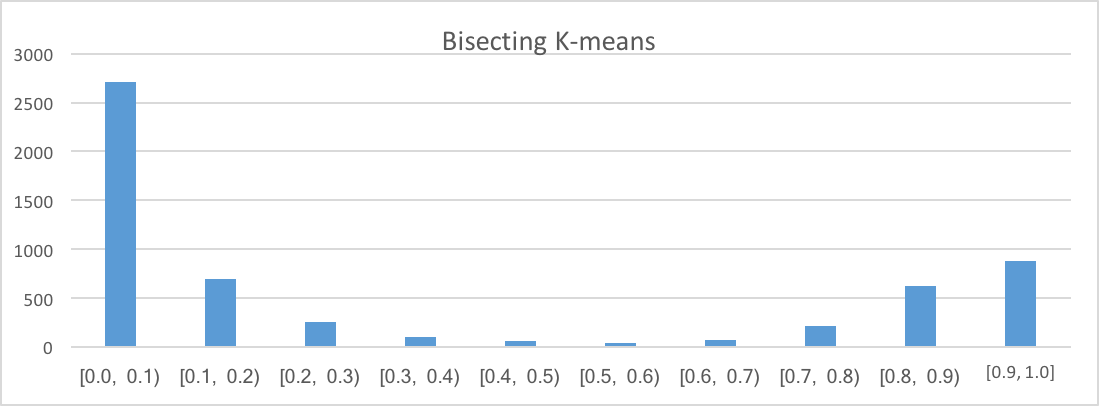Figure 4. Histogram for Bisecting K-means algorithm.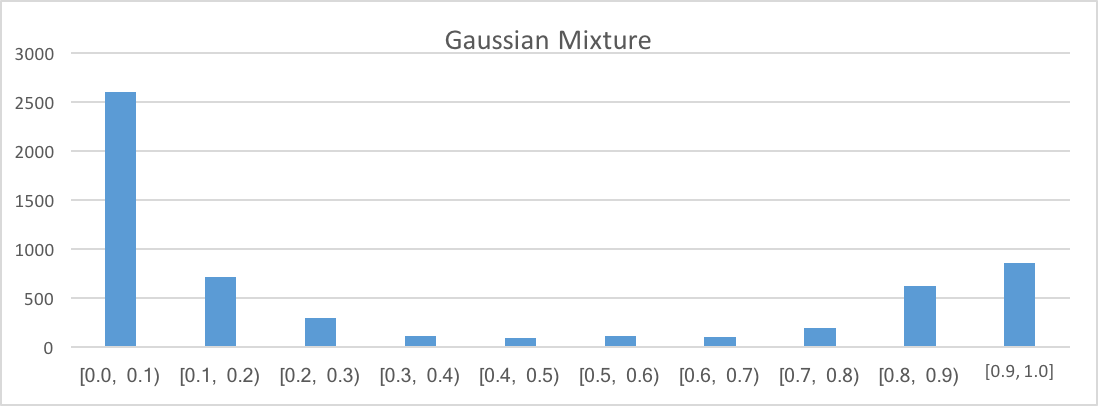Figure 5. Histogram for Gaussian Mixture algorithm.

For Bisecting K-means and Gaussian Mixture algorithms, the data mostly accumulates in the bins near 0 and 1, specifically in the intervals [0,0.2] and [0.8,1.0]. On the other hand, for the K-means algorithm, the bins between 0 and 1 contain far too many data points, particularly in the interval [0.4,0.7]. Therefore, we conclude that the K-means algorithm does not achieve a consensus as satisfactory as Bisecting K-means or Gaussian Mixture for the particular data set.

## Code Review

We implemented a sample Java application to demonstrate consensus clustering. The application uses the Apache Spark machine learning library APIs for K-means, Bisecting K-means, and Gaussian Mixture (Spark version 2.3.0). An abstract class named `ConsensusCluster` stores all the logic for calculating the histogram. Three concrete classes, `BisectingKMeansClustering``KMeansClustering` and, `GaussianMixtureClustering`each representing a particular clustering algorithm, create the actual clusters.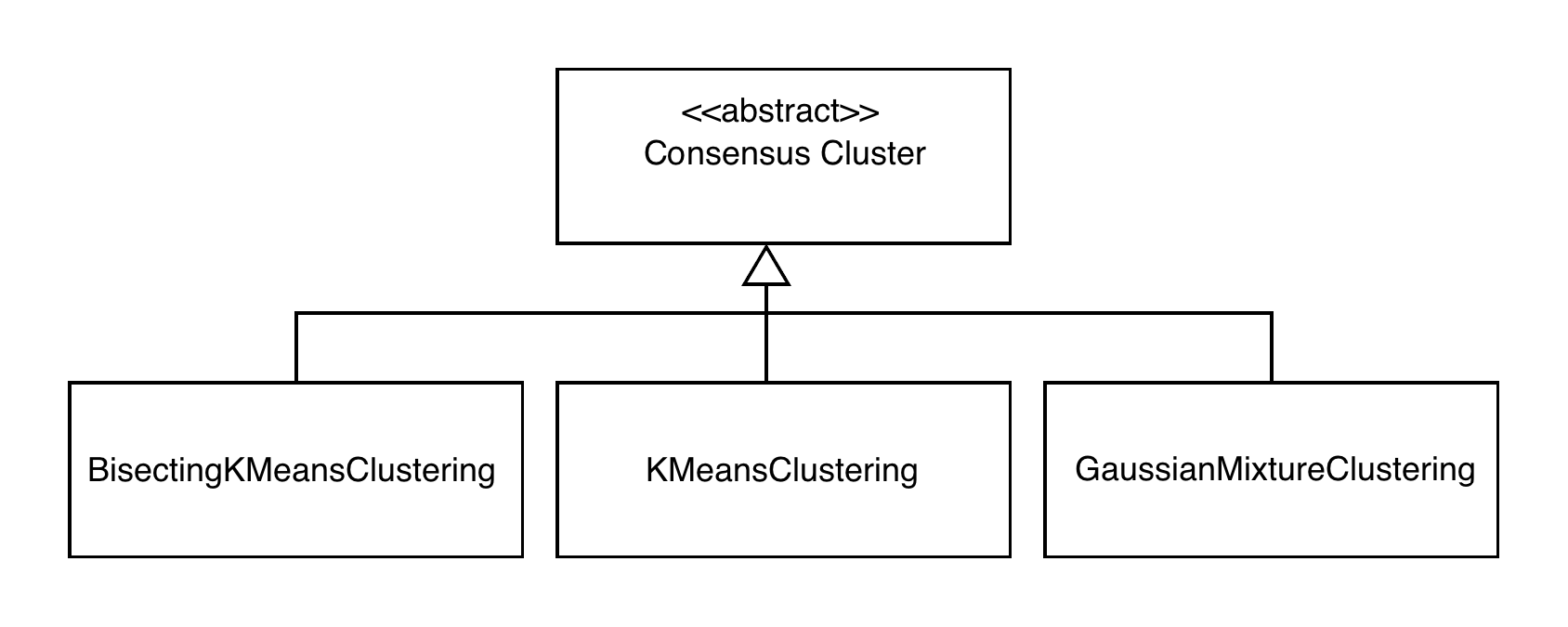Figure 6. Class diagram for the demo application.

The sample application uses a data set, as previously mentioned in 'Problem Statement,' downloaded from National Cancer Institute GDC Data Portal regarding Glioblastoma Multiforme (GBM) and Low-Grade Glioma (LGG) patients . It consists of 76 distinct data points. For consensus calculations, we ran 20 iterations. In each iteration, data was randomly subsampled with each subsample containing ~90% of the original (full) data set.

With each algorithm, we created three clusters in every iteration. We generated histogram data after all iterations are completed. The plots of the histograms for each algorithm are given in Figures 3, 4, 5.

## ConsensusCluster

This parent class performs most of the work for calculating the histogram for consensus indices. The only work delegated to concrete child classes is the creation of clusters.

``````import java.util.ArrayList;
import java.util.HashMap;
import java.util.HashSet;
import java.util.List;
import java.util.Random;
import java.util.Set;
import org.apache.spark.SparkConf;
import org.apache.spark.api.java.JavaRDD;
import org.apache.spark.api.java.JavaSparkContext;
import org.apache.spark.api.java.function.Function;
import org.apache.spark.mllib.linalg.Vector;
import org.apache.spark.mllib.linalg.Vectors;

public abstract class ConsensusCluster{

// Number of cluster we would like to construct
protected static int numClusters = 3;

// Stores how many times two data points were assigned to the same cluster
// across all iterations.
//
// A data point is represented as a Row object. Key to the HashMap is a data point
// (outer point) and the corresponding value is a HashMap that maps a data point
// (inner point) to an integer. Hence, to find how many times two data points were
// assigned to the same cluster across all iterations pass one of those data points
// (outer point) to countSameCluster to get the corresponding HashMap. Then, pass
// the second data point(inner point) to that HashMap to get the integer value,
// which is how many times those data points were assigned to the same cluster.
protected HashMap<String, HashMap<String, Integer>> countSameCluster =
new HashMap<String, HashMap<String, Integer>>();

// Stores how many times two data points were assigned to the same data sample
// across all iterations.
//
// A data point is represented as a Row object. Key to the HashMap is a data point
// (outer point) and the corresponding value is a HashMap that maps a data point
// (inner point) to an integer.Hence, to find how many times two data points were
// in the same data sample across all iterations pass one of those data points
// (outer point) to countSameSample to get the corresponding HashMap. Then, pass
// the second data point (inner point) to that HashMap to get the integer value,
// which is how many times those data points were in the same data sample.
protected HashMap<String, HashMap<String, Integer>> countSameSample =
new HashMap<String, HashMap<String, Integer>>();``````

Those data structures are initialized by the following method.

``````/**
* Initialize 'countSameCluster' & 'countSameCluster' from full data set
*
* @param fullData: full data set
*/
protected void initializeCounts(JavaRDD<String> fullData) {
fullData.cache();
List<String> pointsOuter = fullData.collect();
List<String> pointsInner = fullData.collect();

for (String pointOuter : pointsOuter) {
HashMap<String, Integer> map = new HashMap<String, Integer>();
for (String pointInner : pointsInner) {
map.put(pointInner, 0);
}
countSameCluster.put(pointOuter, map);
}

for (String pointOuter : pointsOuter) {
HashMap<String, Integer> map = new HashMap<String, Integer>();
for (String pointInner : pointsInner) {
map.put(pointInner, 0);
}
countSameSample.put(pointOuter, map);
}
}``````

The following method updates the '`countSameSample`' data structure from a given subsample at a particular iteration.

``````/**
* Given a subsample update the 'countSameSample' data structure.
* @param collectedSampledData - Data points in a subsample
*/
protected void updateSameSampleCounts(List<String> collectedSampledData) {
ArrayList<String> clonedData = new ArrayList<String>();
for (String s : collectedSampledData) {
}

for (String s : collectedSampledData) {
// Get all the points in 'countSameSample' for the particular
// data point s in subsample
HashMap<String, Integer> allPoints = countSameSample.get(s);

for (String c : clonedData) {
if(s.equals(c)){
continue; // ignore self
}
// Increment # times c & s were together in a subsample
int currentVal = allPoints.get(c);
currentVal++;
allPoints.put(c, currentVal);
}
}
}``````

The following method updates the '`countSameCluster`' data structure from data points in a cluster at a particular iteration.

``````/**
* Given a cluster update 'countSameCluster' data structure
* from data points in a cluster
* @param clusterPoints - Data points in a cluster
*/
protected void updateSameClusterCounts(HashMap<Integer, Set<String>> clusterPoints) {
// Each element in keys corresponds to a particular cluster, 0, 1, 2
Set<Integer> keys = clusterPoints.keySet();

for (int i : keys) {
// Obtain points in that cluster
Set<String> pointsOuter = clusterPoints.get(i);
Set<String> pointsInner = clusterPoints.get(i);

for (String pointOuter : pointsOuter) {
// Get all the points in 'countSameCluster' for the
// particular data point pointOuter in cluster
HashMap<String, Integer> allPoints = countSameCluster.get(pointOuter);

for (String pointInner : pointsInner) {
if(pointOuter.equals(pointInner)){
continue; // ignore self
}
// Increment # times pointInner & pointOuter were together in a
// cluster
int currentVal = allPoints.get(pointInner);
currentVal++;
allPoints.put(pointInner, currentVal);
}
}
}
}``````

The following method utilizes the previously introduced methods to update '`countSameSample`' and '`countSameCluster`' at the end of each iteration.

``````/**
* At end of an iteration, update global data structures based on the subsample
* used in the iteration and data points in the clusters created in that
* iteration
*
* @param collectedSampledData - An ordered collection storing data points where
* point at i-th element
* belongs to the cluster defined in i-th element of collectedClusterIndexes
* @param collectedClusterIndexes - An ordered collection where each element
* represents a cluster, 0, 1, 2
*
* @param clusterCenters - Coordinates of each cluster
*/
protected void updateHistogramData(List<String> collectedSampledData,
List<Integer> collectedClusterIndexes,
Vector[] clusterCenters) {

// Update the 'countSameSample' data structure

// Key to 'clusterPoints' is a cluster identifier, e.g. 0, 1, 2
// The value is a Set where each element is a data point in the corresponding
// cluster; data point is represented by its coordinates - a string of
// whitespace separated numbers
HashMap<Integer, Set<String>> clusterPoints = new HashMap<Integer,
Set<String>>();
int j = 0;

for (Integer i : collectedClusterIndexes) {
Set<String> points = clusterPoints.get(i);
if (points == null) {
points = new HashSet<String>();
clusterPoints.put(i, points);
}
String tempRow = collectedSampledData.get(j++);
}

// Update the 'countSameCluster' data structure
}``````

After all the iterations are completed, this method will calculate and print out the histogram data. Then, the histogram can be plotted using a graphical tool such as Microsoft Excel.

``````/**
* Calculate and print out histogram data. Data for 10 bins will be printed out:
* 0.0 <number to display in bin [0.0,0.1)>
* 0.1 <number to display in bin [0.1,0.2)>
* 0.2 <number to display in bin [0.2,0.3)>
* ...
* 0.9 <number to display in bin [0.9,1.0]>
*/
protected void generateHistogram() {
// range: 0.1
HashMap<Integer, Integer> histogram = new HashMap<Integer, Integer>();

// Initialize with all 0's.
histogram.put(0, 0);
histogram.put(1, 0);
histogram.put(2, 0);
histogram.put(3, 0);
histogram.put(4, 0);
histogram.put(5, 0);
histogram.put(6, 0);
histogram.put(7, 0);
histogram.put(8, 0);
histogram.put(9, 0);

for (String sOuter : countSameCluster.keySet()) {
// sOuter is a particular data point.
// inSameCluster stores how many times a particular data point was in
// the same cluster as sOuter
// inSameSample stores how many times a particular data point was in
// the same subsample as sOuter
HashMap<String, Integer> inSameCluster = countSameCluster.get(sOuter);
HashMap<String, Integer> inSameSample = countSameSample.get(sOuter);

for (String sInner : inSameCluster.keySet()) {
// sInner is a particular data point that was in the same cluster as
// sOuter
if (sOuter.equals(sInner))
continue; // Ignore self

// how many times sInner and sOuter were in the same cluster
int numTimesInSameCluster = inSameCluster.get(sInner);

// how many times sInner and sOuter were in the same subsample
int numTimesInSameSample = inSameSample.get(sInner);

// Calculate the ratio and place into the corresponding bin
float ratio = (numTimesInSameCluster == 0) ? 0f :
(float) numTimesInSameCluster / numTimesInSameSample;

if (0 <= ratio && ratio < 0.1) {
int val = histogram.get(0);
val++;
histogram.put(0, val);
} else if (0.1 <= ratio && ratio < 0.2) {
int val = histogram.get(1);
val++;
histogram.put(1, val);
} else if (0.2 <= ratio && ratio < 0.3) {
int val = histogram.get(2);
val++;
histogram.put(2, val);
} else if (0.3 <= ratio && ratio < 0.4) {
int val = histogram.get(3);
val++;
histogram.put(3, val);
} else if (0.4 <= ratio && ratio < 0.5) {
int val = histogram.get(4);
val++;
histogram.put(4, val);
} else if (0.5 <= ratio && ratio < 0.6) {
int val = histogram.get(5);
val++;
histogram.put(5, val);
} else if (0.6 <= ratio && ratio < 0.7) {
int val = histogram.get(6);
val++;
histogram.put(6, val);
} else if (0.7 <= ratio && ratio < 0.8) {
int val = histogram.get(7);
val++;
histogram.put(7, val);
} else if (0.8 <= ratio && ratio < 0.9) {
int val = histogram.get(8);
val++;
histogram.put(8, val);
} else if (0.9 <= ratio && ratio <= 1) {
int val = histogram.get(9);
val++;
histogram.put(9, val);
}
}
}
// Display histogram data
System.out.println("HISTOGRAM");
for (int i : histogram.keySet()) {
System.out.println(String.format("%.1f", (double)(i * 0.1)) + " " +
histogram.get(i));
}
}``````

Below is a helper function that converts a data point, expressed as a string consisting of 3 numbers, into a 3-D vector of doubles.

``````/**
* This is a helper function that converts a data point, expressed as a string
* consisting of 3 numbers,
* into a 3-D vector of doubles.
*/
@SuppressWarnings("serial")
static Function<String, Vector> mapFunction = new Function<String, Vector>() {
public Vector call(String s) {
String[] sarray = s.split(" ");
double[] values = new double[sarray.length];

for (int i = 0; i < sarray.length; i++)
values[i] = Double.parseDouble(sarray[i]);

return Vectors.dense(values);
}
};``````

This is the abstract method to be implemented by child classes. It partitions subsampled data into clusters.

``````/**
* Partition subsampled data into clusters. Implemented by a concrete class based
* on a specific algorithm.
*
* @param data - subsampled data
* @param numClusters - # clusters to create
* @param numIterations - # iterations to perform
* @return JavaRDD<Integer> - This data structure has the same number of elements
* as input parameter
* data. Each element in JavaRDD<Integer> indicates which cluster the corresponding
* element in data belongs to, e.g. 0, 1, 2 etc.
*
*/
protected abstract JavaRDD<Integer> dataCenters(JavaRDD<Vector> data,
int numClusters, int numIterations);``````

This is the main method. It starts Spark configuration and context, initializes the data structures, and starts iterations. In each iteration, it creates clusters via a concrete subclass and updates the data structures from that iteration. Finally, it calculates and prints out the histogram data.

``````/**
* This is the main method that performs all the tasks.
*/
protected void iterations() {
// Set application name
String appName = "Consensus Clustering Demo";

// # iterations to determine clusters
int numIterations = 125;

// Initialize Spark configuration & context
SparkConf sparkConf = new SparkConf().setAppName(appName).
setMaster("local").set("spark.executor.memory",
"1g");

JavaSparkContext sc = new JavaSparkContext(sparkConf);

// Read data file from file system.
String path = "resources/TP53-VarClsf.txt";

// Read the data file and return it as JavaRDD of strings
JavaRDD<String> nonUniqueData = sc.textFile(path);
System.out.println(nonUniqueData.count());

// Remove any duplicates
JavaRDD<String> fullData = nonUniqueData.distinct();
System.out.println(fullData.count());

// Initialize global data structures
initializeCounts(fullData);

// Number of iterations
int MAX_ITER = 20;

// Each execution of this loop corresponds to an iteration
for (int iteration = 0; iteration <= MAX_ITER; iteration++) {
// Obtain a random subsample, consisting of 90% of the original data set
JavaRDD<String> sampledData = fullData.sample(false, 0.9, (new
Random().nextLong()));

// Convert data point, expressed as a string consisting of 3 numbers, into
// a 3-D vector of doubles
JavaRDD<Vector> data = sampledData.map(mapFunction);
data.cache();

// Rely on concrete subclasses for this method. This is where clusters are
// created by a specific algorithm
Tuple2<JavaRDD<Integer>, Vector[]> pair =
dataCenters(data, numClusters, numIterations);

// Each element in clusterIndexes represents a cluster, 0, 1, 2
JavaRDD<Integer> clusterIndexes = pair._1();

// Each element in clusterCenters gives coordinates of a particular
// cluster, 0, 1, 2
Vector[] clusterCenters = pair._2();

// Bring all data to driver node for displaying results.
// 'collectedSampledData' and 'collectedClusterIndexes' are ordered
// collections with the same size: i-th element in 'collectedSampledData'
// is a data point that belongs to the cluster defined in the i-th element
// in 'collectedClusterIndexes'
List<String> collectedSampledData = sampledData.collect();
List<Integer> collectedClusterIndexes = clusterIndexes.collect();

System.out.println(collectedSampledData.size() + " " +
collectedClusterIndexes.size());

// Update global data structures based on cluster data
updateHistogramData(collectedSampledData, collectedClusterIndexes,
clusterCenters);
}

// Generate histogram
generateHistogram();

// Stop spark
sc.stop();
sc.close();
}``````

Now, we will review the child classes. Each child class will implement protected abstract JavaRDD<Integer> dataCenters() to partition subsampled data into clusters based on a specific algorithm.

## KMeansClustering

In its main method, `KMeansClustering` triggers `iterations()` implemented by the parent class. It also implements the `dataCenters()` method to create clusters based on the K-means algorithm using KMeans and KMeansModel in the org.apache.spark.mllib.clustering package.

``````import org.apache.log4j.Level;
import org.apache.log4j.Logger;
import org.apache.spark.api.java.JavaRDD;
import org.apache.spark.mllib.clustering.KMeans;
import org.apache.spark.mllib.clustering.KMeansModel;
import org.apache.spark.mllib.linalg.Vector;

public class KMeansClustering extends ConsensusCluster {

/**
* The main method. It triggers iterations() implemented by the
* parent class.
*/
public static void main(String[] args) throws Exception {
Logger.getRootLogger().setLevel(Level.WARN);
(new KMeansClustering()).iterations();
}

/**
* In ConsensusCluster, this method was abstract; implementation
* was left to child classes. Here we first create a KMeans object and then
* call its run() method on data to obtain a KMeansModel object. Then, we call
* the predict() method on KMeansModel object to obtain a data structure,
* clusterIndexes, that gives the cluster indexes for the input parameter
* data. The clusterIndexes and data have the same number of elements. The
* elements in the clusterIndexes and data have reciprocal sequence in the
* sense that the i-th element of clusterIndexes defines which cluster the
* i-th element of data belongs to, one of 0, 1, 2 ... etc.
*
* @param data - Data points to partition into clusters
* @param numClusters - number of clusters desired
* @param numIterations - maximum # of iterations to perform during clustering
*
*/
public JavaRDD<Integer> dataCenters(JavaRDD<Vector> data, int numClusters,
int numIterations){
KMeans km = new
KMeans().setK(numClusters).setMaxIterations(numIterations);
KMeansModel clusters = km.run(data.rdd());
JavaRDD<Integer> clusterIndexes = clusters.predict(data);
return clusterIndexes;
}
}``````

## BisectingKMeansClustering

In its main method, `BisectingKMeansClustering` triggers `iterations()` implemented by the parent class. It also implements the `dataCenters()` method to create clusters based on the Bisecting K-means algorithm using BisectingKMeans and BisectingKMeansModel in the org.apache.spark.mllib.clustering package.

``````import org.apache.log4j.Level;
import org.apache.log4j.Logger;
import org.apache.spark.api.java.JavaRDD;
import org.apache.spark.mllib.clustering.BisectingKMeans;
import org.apache.spark.mllib.clustering.BisectingKMeansModel;
import org.apache.spark.mllib.linalg.Vector;

public class BisectingKMeansClustering extends ConsensusCluster{

/**
* The main method. It triggers iterations() implemented by the
* parent class.
*/
public static void main(String[] args) throws Exception {
Logger.getRootLogger().setLevel(Level.WARN);
(new BisectingKMeansClustering()).iterations();
}

/**
* In ConsensusCluster, this method was abstract; implementation
* was left to child classes. Here we first create a BisectingKMeans object
* and then call its run() method on data to obtain a BisectingKMeansModel
* object. Then, we call the predict() method on BisectingKMeansModel object
* to obtain a data structure, clusterIndexes, that gives the cluster indexes
* for the input parameter data. The clusterIndexes and data have the same
* number of elements. The elements in the clusterIndexes and data have
* reciprocal sequence in the sense that the i-th element of clusterIndexes
* defines which cluster the i-th element of data belongs to, one of 0, 1,
* 2 ... etc.
*
* @param data - Data points to partition into clusters
* @param numClusters - number of clusters desired
* @param numIterations - maximum # of iterations to perform during clustering
*
*/
public JavaRDD<Integer> dataCenters(JavaRDD<Vector> data, int numClusters,
int numIterations){
BisectingKMeans bkm = new
BisectingKMeans().setK(numClusters).setMaxIterations(numIterations);
BisectingKMeansModel clusters = bkm.run(data);
JavaRDD<Integer> clusterIndexes = clusters.predict(data);
return clusterIndexes;
}
}``````

## GaussianMixtureClustering

In its main method, `GaussianMixtureClustering` triggers `iterations()` implemented by the parent class. It also implements the `dataCenters()` method to assign data points to clusters based on Gaussian Mixture algorithm using GaussianMixture and GaussianMixtureModel in the org.apache.spark.mllib.clustering package.

``````import org.apache.log4j.Level;
import org.apache.log4j.Logger;
import org.apache.spark.api.java.JavaRDD;
import org.apache.spark.mllib.clustering.GaussianMixture;
import org.apache.spark.mllib.clustering.GaussianMixtureModel;
import org.apache.spark.mllib.linalg.Vector;

public class GaussianMixtureClustering extends ConsensusCluster {

/**
* The main method. It triggers iterations() implemented by the
* parent class.
*/
public static void main(String[] args) throws Exception {
Logger.getRootLogger().setLevel(Level.WARN);
(new BisectingKMeansClustering()).iterations();
}

/**
* In ConsensusCluster, this method was abstract; implementation
* was left to child classes. Here we first create a GaussianMixture object
* and then call its run() method on data to obtain a GaussianMixtureModel
* object. Then, we call the predict method on GaussianMixtureModel object to
* obtain a data structure, clusterIndexes, that gives the cluster indexes for
* the input parameter data. The clusterIndexes and data have the same number
* of elements. The elements in the clusterIndexes and data have reciprocal
* sequence in the sense that the i-th element of clusterIndexes
* defines which cluster the i-th element of data belongs to, one of 0, 1,
* 2 ... etc.
*
* @param data - Data points to partition into clusters
* @param numClusters - number of clusters desired
* @param numIterations - maximum # of iterations to perform during clustering
*
*/
protected JavaRDD<Integer> dataCenters(JavaRDD<Vector> data, int numClusters,
int numIterations) {
GaussianMixture gm = new
GaussianMixture().setK(numClusters).setMaxIterations(numIterations);
GaussianMixtureModel clusters = gm.run(data.rdd());
JavaRDD<Integer> clusterIndexes = clusters.predict(data);
return clusterIndexes;
}
}``````

## Conclusions

In this article, we discussed consensus clustering, a technique to represent consensus across multiple runs of a clustering algorithm and analyze the stability of discovered clusters with respect to sampling variability. We reviewed a sample application built with the Apache Spark machine learning library to show how consensus clustering can be used with K-means, Bisecting K-means, and Gaussian Mixture, three distinct clustering algorithms. For the example data set considered in the application, we calculated and plotted the histograms of consensus indices. The histograms indicated that Bisecting K-means and Gaussian Mixture algorithms delivered more stable clusters than the K-means algorithm. Some concluding remarks are as follows.

• Consensus clustering can be applied to any clustering algorithm and is useful to compare different algorithms on the same dataset. However, consensus clustering does not aim at deciding which clustering algorithm is more stable than other algorithms in general. The results are dependent on a particular data set and the nature of the relationship between data points. For example, a hierarchical clustering algorithm may fail to achieve satisfactory consensus for a data set that is not hierarchical in nature, or vice versa.

• Consensus clustering can additionally be used to determine the optimal number of clusters for a data set and clustering algorithm. A consensus matrix could also be used as a similarity measure, an alternative to other measures, e.g. Euclidean distance .

• The 3-dimensional graphs of sample clusters in Figures 1.a, 1.b were obtained by Java-based jzy3d framework  and histograms in Figures 3 – 5 were plotted by Microsoft Excel.

• Complete source code for the sample application can be found in .

# References

 Cluster Analysis, Wikipedia.

 Automatic symmetry based cluster approach for anomalous brain identification in PET scan image – An Analysis, A. Meena and K. Raja, Cornell University Library.

 Data Clustering Using Apache Spark, K. Unyelioglu, DZone / Big Data Zone.

 Classification and Prediction of Survival in Hepatocellular Carcinoma by Gene Expression Profiling, J-S Lee et. al., Hepatology, 2004.

 The Elements of Statistical Learning, T. Hastie et. al., Springer Series in Statistics, 2009.

 Pattern Classification, R.O. Duda et. al., John Wiley & Sons, 2000.

 Consensus Clustering: A Resampling-Based Method for Class Discovery and Visualization of Gene Expression Microarray Data, S. Monti et. al., Machine Learning, 52, 91–118, 2003.

 Apache Spark, spark.apache.org.

 Heat Map, Wikipedia.

 The History of the Cluster Heat Map, L. Wilkinson and M. Friendly, The American Statistician Volume 63 Issue 2, 2009.

 National Cancer Institute Genomic Data Commons Project, gdc.cancer.gov.

 Open Source API for 3D Charts, jzy3d.org.

 Consensus-clustering, github.com.

Cloudera Enterprise Data Hub. One platform, many applications. Start today.

Topics:
cluster analysis ,apache spark ,apache spark examples java ,k-means clustering ,big data

Comment (0)

Save
{{ articles.views | formatCount}} Views

Opinions expressed by DZone contributors are their own.

# {{ parent.title || parent.header.title}}

{{ parent.tldr }}

{{ parent.urlSource.name }}